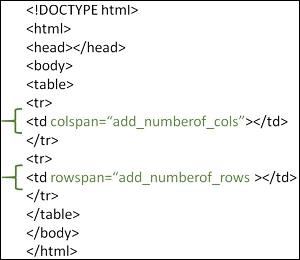# What are table rowspan and colspan in HTML?

The rowspan and colspan are the attributes of <td> tag. These are used to specify the number of rows or columns a cell should merge. The rowspan attribute is for merging rows and the colspan attribute is for merging columns of the table in HTML.

These attributes should be placed inside the <td> tag as shown in the image given below −## Syntax

Following is the syntax to merge table cells in HTML

<td rowspan="2">cell data</td>
<td colspan="2">cell data</td>


### Example 1 − Setting the rowspan

Now let us look at an example where we set the rowspan of the one of the columns to 2.

<!DOCTYPE html>
<html>
<meta charset="UTF-8">
<meta http-equiv="X-UA-Compatible" content="IE=edge">
<meta name="viewport" content="width=device-width, initial-scale=1.0">
<style>
table,
tr,
th,
td {
border: 1px solid black;
}
</style>
<body>
<h2>Tables in HTML</h2>
<table style="width: 100%">
<tr>
<th>First Name </th>
<th>Job role</th>
</tr>
<tr>
<td></td>
<td rowspan="2"></td>
</tr>
<tr>
<td></td>
</tr>
<tr>
<td></td>
<td></td>
</tr>
</table>
</body>
</html>


Following is the output for the above example program

### Example 2 − Setting the colspan

Given below is an example to merge column cells of the table in HTML.

<!DOCTYPE html>
<html>
<style>
table,tr,th,td {
border:1px solid black;
}
</style>
<body>
<h2>Tables in HTML</h2>
<table style="width: 100%">
<tr>
<th >First Name </th>
<th>Job role</th>
</tr>
<tr>
<td colspan="2" ></td>
</tr>
<tr>
<td ></td>
<td></td>
</tr>
<tr>
<td colspan="2"></td>
</tr>
</table>
</body>
</html>


Following is the output for the above example program.

### Example 3

Following is another example where merge rows and columns by setting values of both rowspan and colspan attributes in a single program

<html>
<style>
table, th, td {
border: 1px solid black;
width: 100px;
height: 50px;
}
</style>
<body>
<table>
<tr>
<td colspan="2" ></td>
</tr>
<tr>
<td ></td>
<td></td>
</tr>
<tr>
<td colspan="2"></td>
</tr>
</table>
</body>
</html>


Following is the output for the above example program.

Updated on: 06-Sep-2023

33K+ Views